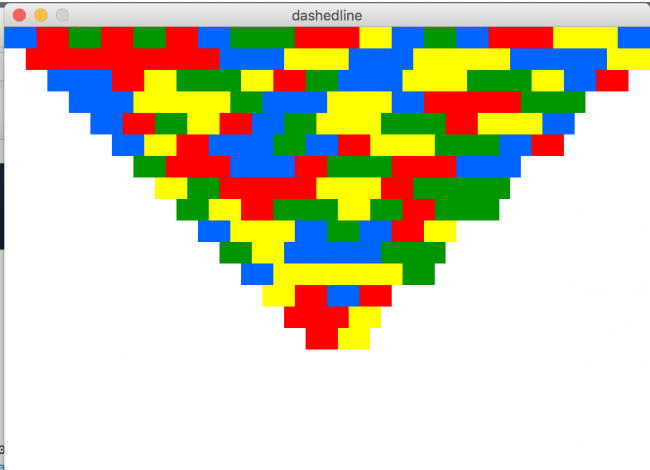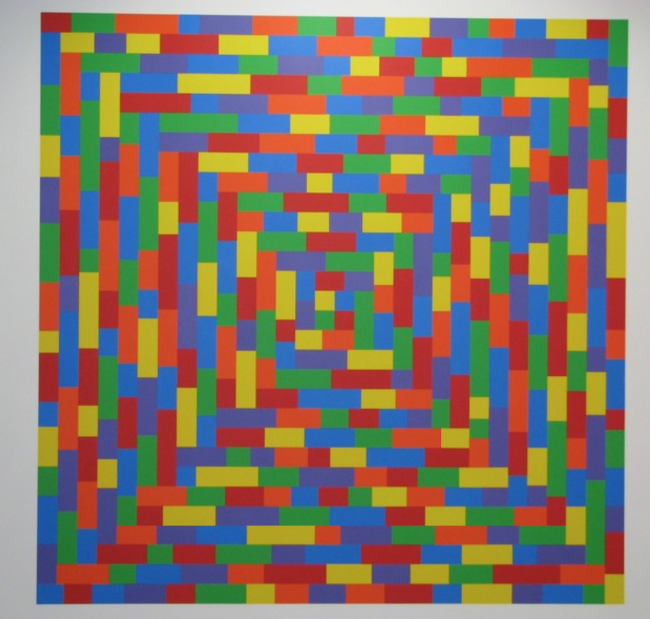Howdy, Stranger!

We are about to switch to a new forum software. Until then we have removed the registration on this forum.

dashed line, max length

edited March 2018

Dear all,

For the last days I've been puzzling on coding this work of lego-ish birdview pyramid.

What I have now is almost good, but the class I'm using to create dashed lines (with colors from an array), are variable length, because it's not limited.

here's the code:

``````        int[] numbers = { 30, 60, 30, 0, 30 };

color [] colarray = {
color(0, 100, 255),
color(255, 255, 0),
color(255, 0, 0),
color(0, 150, 0)};

int col;

void setup( )
{

size(600, 600, P2D);
background(255);
smooth();
strokeCap(SQUARE);
}

void draw( ) {

background(255);
strokeWeight(20);

// top part
for (int i = 0; i < height/2; i = i+20) {
float [ ] dashes = { numbers[(int)random(numbers.length)], numbers[(int)random(numbers.length)], numbers[(int)random(numbers.length)], numbers[(int)random(numbers.length)], numbers[(int)random(numbers.length)]};
dashline(0+i, i+10, width-i, i+10, dashes);
}

// left part
for (int i = 0; i < width/2; i = i+20) {
float [ ] dashes = { numbers[(int)random(numbers.length)], numbers[(int)random(numbers.length)], numbers[(int)random(numbers.length)], numbers[(int)random(numbers.length)], numbers[(int)random(numbers.length)]};
dashline(i+10, 0+i+20, i+10, height-i-20, dashes);
}

// bottom part
for (int i = 0; i < height/2; i = i+20) {
float [ ] dashes = { numbers[(int)random(numbers.length)], numbers[(int)random(numbers.length)], numbers[(int)random(numbers.length)], numbers[(int)random(numbers.length)], numbers[(int)random(numbers.length)]};
dashline(0+i, height-i-10, width-i, height-i-10, dashes);
}

// right part
for (int i = 0; i < width/2; i = i+20) {
float [ ] dashes = { numbers[(int)random(numbers.length)], numbers[(int)random(numbers.length)], numbers[(int)random(numbers.length)], numbers[(int)random(numbers.length)], numbers[(int)random(numbers.length)]};
dashline(width-i+10, 0+i, width-i+10, height-i-20, dashes);
}
}

void dashline(float x0, float y0, float x1, float y1, float[ ] spacing)
{
float distance = dist(x0, y0, x1, y1);
float [ ] xSpacing = new float[spacing.length];
float [ ] ySpacing = new float[spacing.length];
float drawn = 0.0;

if (distance > 0)
{
int i;
boolean drawLine = true;

for (i = 0; i < spacing.length; i++)
{
xSpacing[i] = lerp(0, (x1 - x0), spacing[i] / distance);
ySpacing[i] = lerp(0, (y1 - y0), spacing[i] / distance);
}

i = 0;
while (drawn < distance)
{
col = color(colarray[(int)random(0, 4)]);

stroke(col);

if (drawLine)
{
line(x0, y0, x0 + xSpacing[i], y0 + ySpacing[i]);
} else {
line(x0, y0, x0 + xSpacing[i], y0 + ySpacing[i]);
}

x0 += xSpacing[i];
y0 += ySpacing[i];

drawn = drawn + mag(xSpacing[i], ySpacing[i]);
i = (i + 1) % spacing.length;
drawLine = !drawLine;  // switch between dash and gap
}
}
}
``````

What you see in the next pic is the problem:The right diagonal doesn't make a straight line, because the ending sometimes has picks the next values: int[] numbers = { 30, 60, 30, 0, 30 }; . That is what I want, but I want the line to end no matter what number I use. If the required space is 20 then, 60 should be limited to 20.

Hope someone could give a tip.

• Edit post, highlight code, press ctrl-o to format

• It's not working.

• You can edit your post with the gear

Select entire code with the mouse

Click the small C in the command bar then

• Thanks! :-)

• I didn't fix your code, but sort of made it work. I translate to the center as you need to do the rotation at the center point of your sketch (In case you are wondering). More info: https://processing.org/tutorials/transform2d/

Kf

``````int[] numbers = { 30, 60, 30, 0, 30 };

color [] colarray = {
color(0, 100, 255),
color(255, 255, 0),
color(255, 0, 0),
color(0, 150, 0)};

int col;

void setup( )
{

size(600, 600, P2D);
background(255);
smooth();
strokeCap(SQUARE);

background(255);
strokeWeight(20);
}

void draw( ) {
translate(width/2, height/2);
rotate(HALF_PI);
rotate(HALF_PI);
rotate(HALF_PI);
}

pushMatrix();
translate(-width/2, -height/2);
// top part
for (int i = 0; i < height/2; i = i+20) {
float [ ] dashes = { numbers[(int)random(numbers.length)], numbers[(int)random(numbers.length)], numbers[(int)random(numbers.length)], numbers[(int)random(numbers.length)], numbers[(int)random(numbers.length)]};
dashline(0+i, i+10, width-i, i+10, dashes);
}
popMatrix();
}

void dashline(float x0, float y0, float x1, float y1, float[ ] spacing)
{
float distance = dist(x0, y0, x1, y1);
float [ ] xSpacing = new float[spacing.length];
float [ ] ySpacing = new float[spacing.length];
float drawn = 0.0;

if (distance > 0)
{
int i;
boolean drawLine = true;

for (i = 0; i < spacing.length; i++)
{
xSpacing[i] = lerp(0, (x1 - x0), spacing[i] / distance);
ySpacing[i] = lerp(0, (y1 - y0), spacing[i] / distance);
}

i = 0;
while (drawn < distance)
{
col = color(colarray[(int)random(0, 4)]);

stroke(col);

if (drawLine)
{
line(x0, y0, x0 + xSpacing[i], y0 + ySpacing[i]);
} else {
line(x0, y0, x0 + xSpacing[i], y0 + ySpacing[i]);
}

x0 += xSpacing[i];
y0 += ySpacing[i];

drawn = drawn + mag(xSpacing[i], ySpacing[i]);
i = (i + 1) % spacing.length;
drawLine = !drawLine;  // switch between dash and gap
}
}
}
``````
• If the required space is 20 then, 60 should be limited to 20.

Translate that into code! If have a variable goal, a variable progress you can make this work!

`if(nextline>goal-progress) nextline = goal-progress;`

• Thanks for the help! I found an easy solution. Didn't realize the strokeweight wasn't corresponding with the lenght of the line. In other words: I fixed the values and added some cleverness with global variables.

``````int strokeweight = 20;

int[] numbers = { strokeweight*2, strokeweight*3, strokeweight*2, 0, strokeweight*4 };

int strokeweighthalf = strokeweight/2;

color [] colarray = {
color(0, 100, 255),
color(255, 255, 0),
color(255, 0, 0),
color(0, 150, 0)};

int col;

void setup( )
{

size(600, 600, P2D);
background(255);
smooth();
strokeCap(SQUARE);

background(255);
strokeWeight(strokeweight);
}

void draw( ) {
translate(width/2, height/2);
rotate(HALF_PI);
rotate(HALF_PI);
rotate(HALF_PI);
}

pushMatrix();
translate(-width/2, -height/2);
// top part
for (int i = 0; i < height/2; i = i+strokeweight) {
float [ ] dashes = { numbers[(int)random(numbers.length)], numbers[(int)random(numbers.length)], numbers[(int)random(numbers.length)], numbers[(int)random(numbers.length)], numbers[(int)random(numbers.length)]};
float [ ] dashes2 = { strokeweight};

dashline(0+i, i+strokeweighthalf, width-i-strokeweight, i+strokeweighthalf, dashes);

if ( i > (height/2)-strokeweighthalf) {

dashline(0+i, i+strokeweighthalf, width-i, i+strokeweighthalf, dashes2);
}
}
popMatrix();
}

void dashline(float x0, float y0, float x1, float y1, float[ ] spacing)
{
float distance = dist(x0, y0, x1, y1);
float [ ] xSpacing = new float[spacing.length];
float [ ] ySpacing = new float[spacing.length];
float drawn = 0.0;

if (distance > 0)
{
int i;
boolean drawLine = true;

for (i = 0; i < spacing.length; i++)
{
xSpacing[i] = lerp(0, (x1 - x0), spacing[i] / distance);
ySpacing[i] = lerp(0, (y1 - y0), spacing[i] / distance);
}

i = 0;
while (drawn < distance)
{
col = color(colarray[(int)random(0, 4)]);

stroke(col);

if (drawLine)
{
line(x0, y0, x1, y0 + ySpacing[i]);
} else {
line(x0, y0, x1, y0 + ySpacing[i]);
}

x0 += xSpacing[i];
y0 += ySpacing[i];

drawn = drawn + mag(xSpacing[i], ySpacing[i]);
i = (i + 1) % spacing.length;
drawLine = !drawLine;  // switch between dash and gap
}
}
}
``````
• original image, later removed